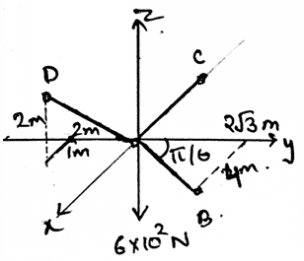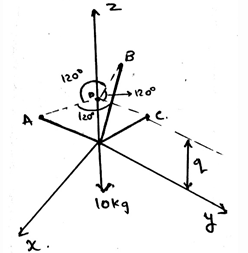Courses

# Test: Cables Subjected To Its Own Weight

## 15 Questions MCQ Test Engineering Mechanics | Test: Cables Subjected To Its Own Weight

Description
This mock test of Test: Cables Subjected To Its Own Weight for Mechanical Engineering helps you for every Mechanical Engineering entrance exam. This contains 15 Multiple Choice Questions for Mechanical Engineering Test: Cables Subjected To Its Own Weight (mcq) to study with solutions a complete question bank. The solved questions answers in this Test: Cables Subjected To Its Own Weight quiz give you a good mix of easy questions and tough questions. Mechanical Engineering students definitely take this Test: Cables Subjected To Its Own Weight exercise for a better result in the exam. You can find other Test: Cables Subjected To Its Own Weight extra questions, long questions & short questions for Mechanical Engineering on EduRev as well by searching above.
QUESTION: 1

### What is not the condition for the equilibrium in three dimensional system of axis for cable subjected to its own weight?

Solution:

For the equilibrium in the three dimensional system of axis we have all the conditions true as, ∑Fx=0, ∑Fy=0 and ∑Fz=0. Also we have the summation of the forces equal to zero. Which is not a non-zero value.

QUESTION: 2

### The assumptions for the calculations are done for the cable subjected to its own weight. In that one of the assumption is that the cable is ___________

Solution:

The assumptions are done so as to make the calculations easy. Though the assumptions make the small errors to not count over the big dat. But still if the assumptions are made then the calculations are easy. Thus the assumption taken as the cable is perfectly flexible.

QUESTION: 3

### The magnitude of the resultant of the two vectors in calculations for cable subjected to its own weight is always:

Solution:

Yes, the magnitude of the resultant of the two vectors always depends on the angle between them. It might be greater or smaller than one of the vector’s length. For perfectly saying, it does depends upon the angle between them.

QUESTION: 4

In the diagram given below, coordinates of D is (1, -2, 2), C (-2, 0, 0) and B are as shown. The dark region is the cables holding the weight of 600N at origin. Find the tension in the AD section.Solution:

As the system is in equilibrium so we need to balance the forces. So when apply the condition of net force to be zero in the z direction, we get (2/3)FAD = 600N. This gives us force along AD be 900N.

QUESTION: 5

Cable subjected to its own weight takes a shape of a ____________ when is subjected to loadings.

Solution:

The cable takes the shape of straight line when subjected to the loadings. As the loadings are straight, acting vertically downwards, they stretch the cables and then make them come in the shape of the straight line. Thus the loadings make the cables come in the straight line.

QUESTION: 6

The force on the cable subjected to its own weight is not neglected in the calculations of the load distribution.

Solution:

The cable is a support system which is used to transfer the loadings in the different structures. The main motto is to make the structure stable. In calculation the self-weight of the cable is neglected and the load is calculated. The forces are acting in the vertically downward direction.

QUESTION: 7

Determine the value of the q, parallel to the z axis. That is the point of intersection of the projections of the points A, B and C parallel to the xy plane. With the distance between the tri-section point and the points A, B and C be equal to 0.6m.Solution:

The application of the equilibrium equation will yield the result. That is the resultant along the z-axis will remain zero. Which give the value of γ as 50˚. And therefore q=51.9cm.

QUESTION: 8

The assumptions for the calculations are done for the cable subjected to its own weight. In that one of the assumption is that the cable is flexible and the other is that the cable is ___________.

Solution:

The assumptions are done so as to make the calculations easy. Though the assumptions make the small errors to not count over the big dat. But still if the assumptions are made then the calculations are easy. Thus the assumption taken as the cable is inextensible.

QUESTION: 9

Due to which property the cable subjected to its own weight, it offers no resistance to bending?

Solution:

Due to the flexibility property the cable, it offers no resistance to bending. As the bending is seen in the beams and all the solid structures. Thus the bending moment produced in the cables are not affecting the cables much. Thus no affect by bending, i.e. no resistance to bending.

QUESTION: 10

The tensile force acting on the cable subjected to its own weight is in which direction w.r.t the cable?

Solution:

Due to the flexibility property the cable, it offers no resistance to bending. As the bending is seen in the beams and all the solid structures. Thus the bending moment produced in the cables are not affecting the cables much. So the tensile force which is being produced is acting in the tangential direction to the points of the cable along its lengths.

QUESTION: 11

The cable weight become significant in the calculations of the loadings when the cable subjected to its own weight are used in the transmission lines and guys for radio antennas.

Solution:

The cable is a support system which is used to transfer the loadings in the different structures. The main motto is to make the structure stable. But in the calculations of the loadings when the cables are used in the transmission lines and guys for antennas the self-weight of the cable is not neglected and the load is calculated.

QUESTION: 12

The loading in the cable subjected to its own weight doesn’t changes the ___________ of the cables.

Solution:

The loading in the cables doesn’t affect the geometry of the cables. This is because of the assumptions which we have taken. The first one that the cables are perfectly elastic. And the second one that the cables are inextensible.

QUESTION: 13

If the unknown variables in the calculations are more than the known quantities, then the number of equations required to solve all the unknown variables are for cable subjected to its own weight?

Solution:

Whatever be the calculation involved, the unknown variables will be ultimately come out from the equations. The proper use of the known quantities and the multiuse of various calculation techniques will ultimately give up the results. Thus whether it may be the case of the cables or the beams the equations if applied properly will result in the determination of the unknowns.

QUESTION: 14

For calculations involved for cable subjected to its own weight all the vectors quantities obey :

Solution:

All the vectors quantities obey parallelogram law of addition. Two vectors A and B (can be called as component vectors) are added to form a resultant vector. R = A+B.

QUESTION: 15

If two equal vector forces are mutually perpendicular in the cable subjected to its own weight then the resultant force is acting at which angle as compared to one of the vector?

Solution:

The vectors are mutually perpendicular, this means that the angle between the forces is 90 degree. Thus the resultant will form at 45 degrees to any of the vector.